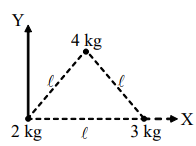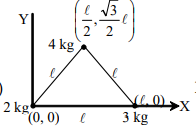Location of centre on mass for multiple point masses
Question

# Three masses 2 kg, 3 kg and 4 kg are lying at the corners of an equilateral triangle of side $\ell$.The (X)coordinate of center of mass isModerate
Solution

##${\mathrm{X}}_{\mathrm{cm}}=\frac{{\mathrm{m}}_{1}{\mathrm{x}}_{1}+{\mathrm{m}}_{2}{\mathrm{x}}_{2}+{\mathrm{m}}_{3}{\mathrm{x}}_{3}}{{\mathrm{m}}_{1}+{\mathrm{m}}_{2}+{\mathrm{m}}_{3}}=\frac{2×0+3×\mathrm{\ell }+4×\frac{\mathrm{\ell }}{2}}{2+3+4}=\frac{5}{9}\mathrm{\ell }$

Get Instant Solutions